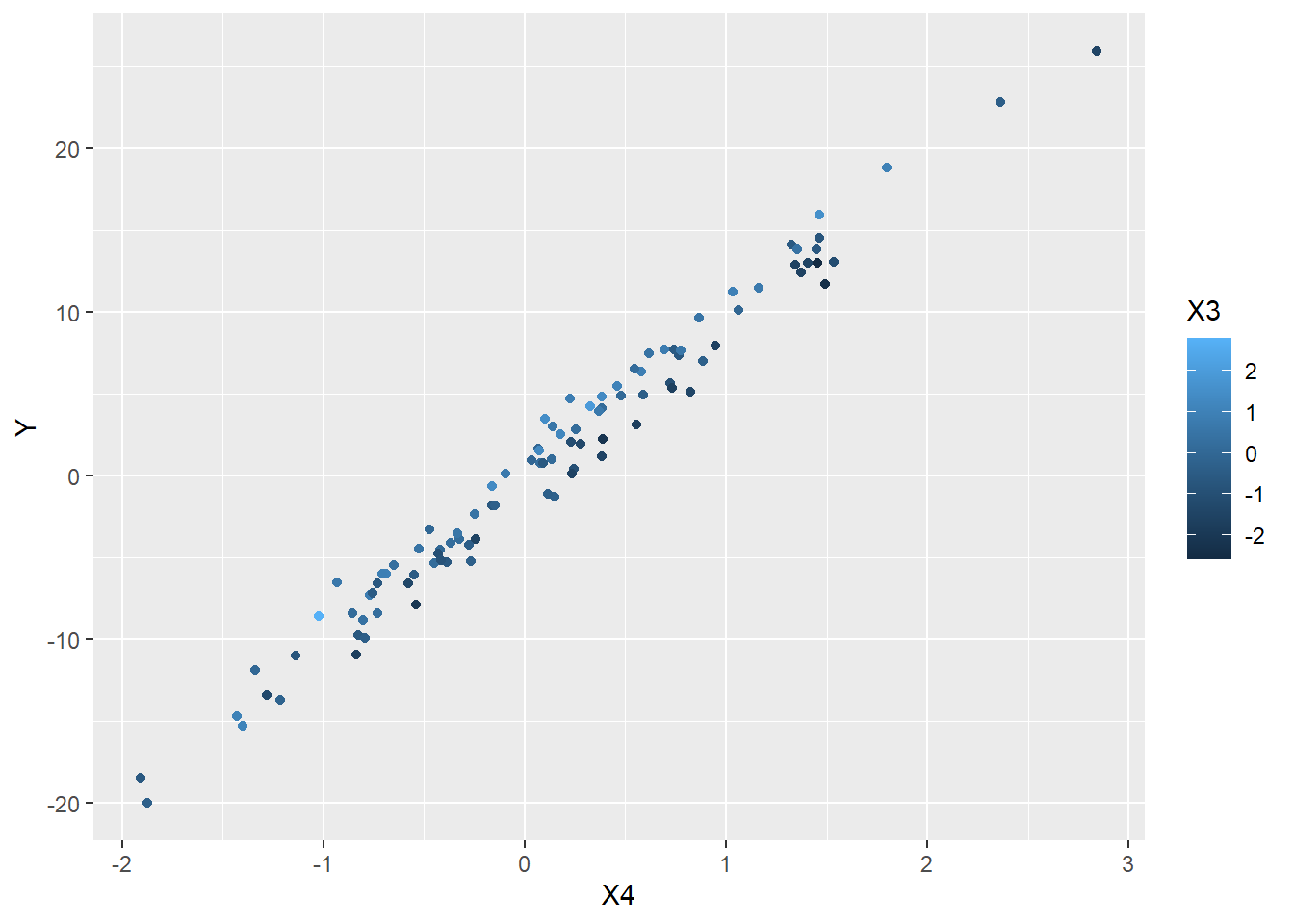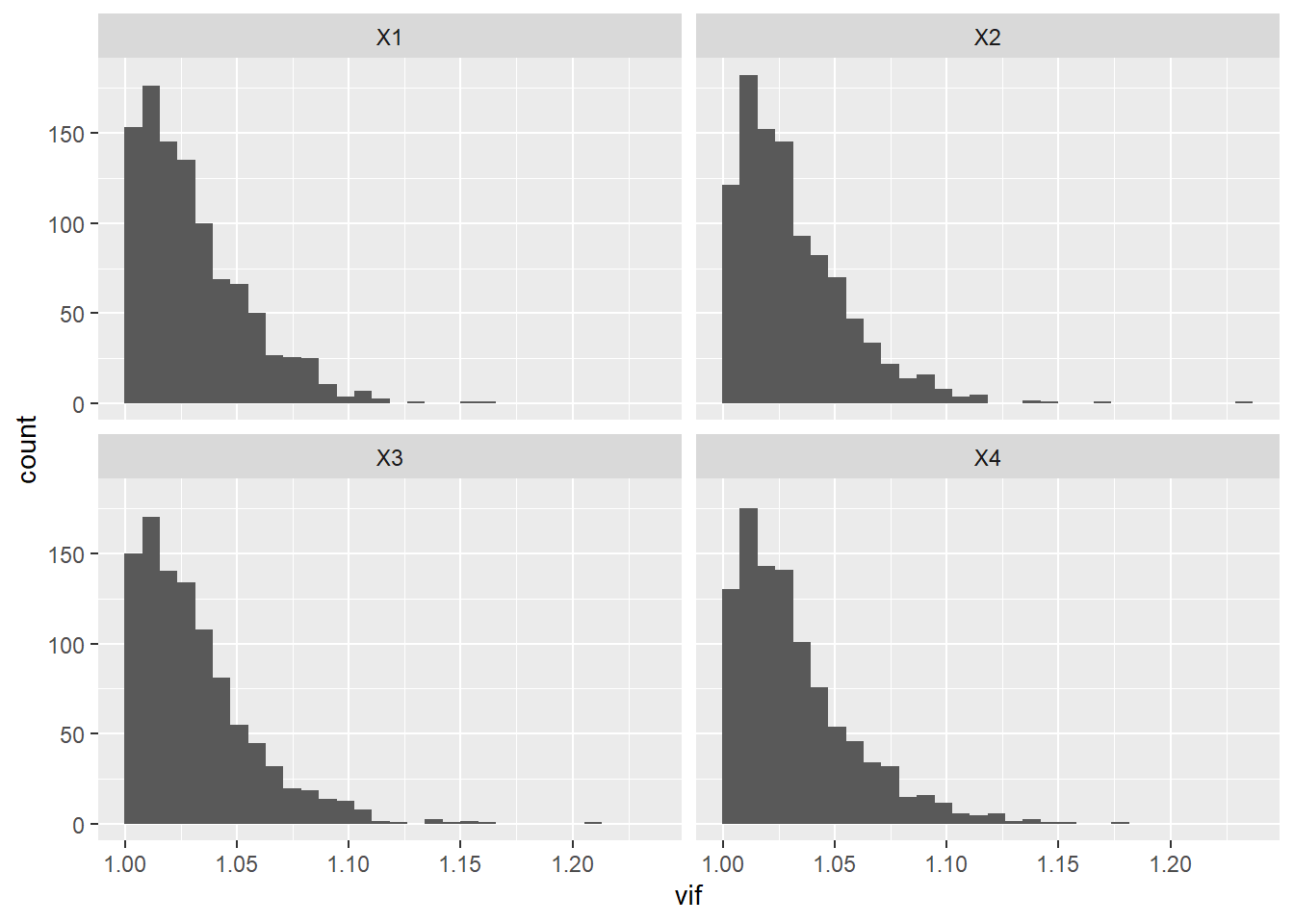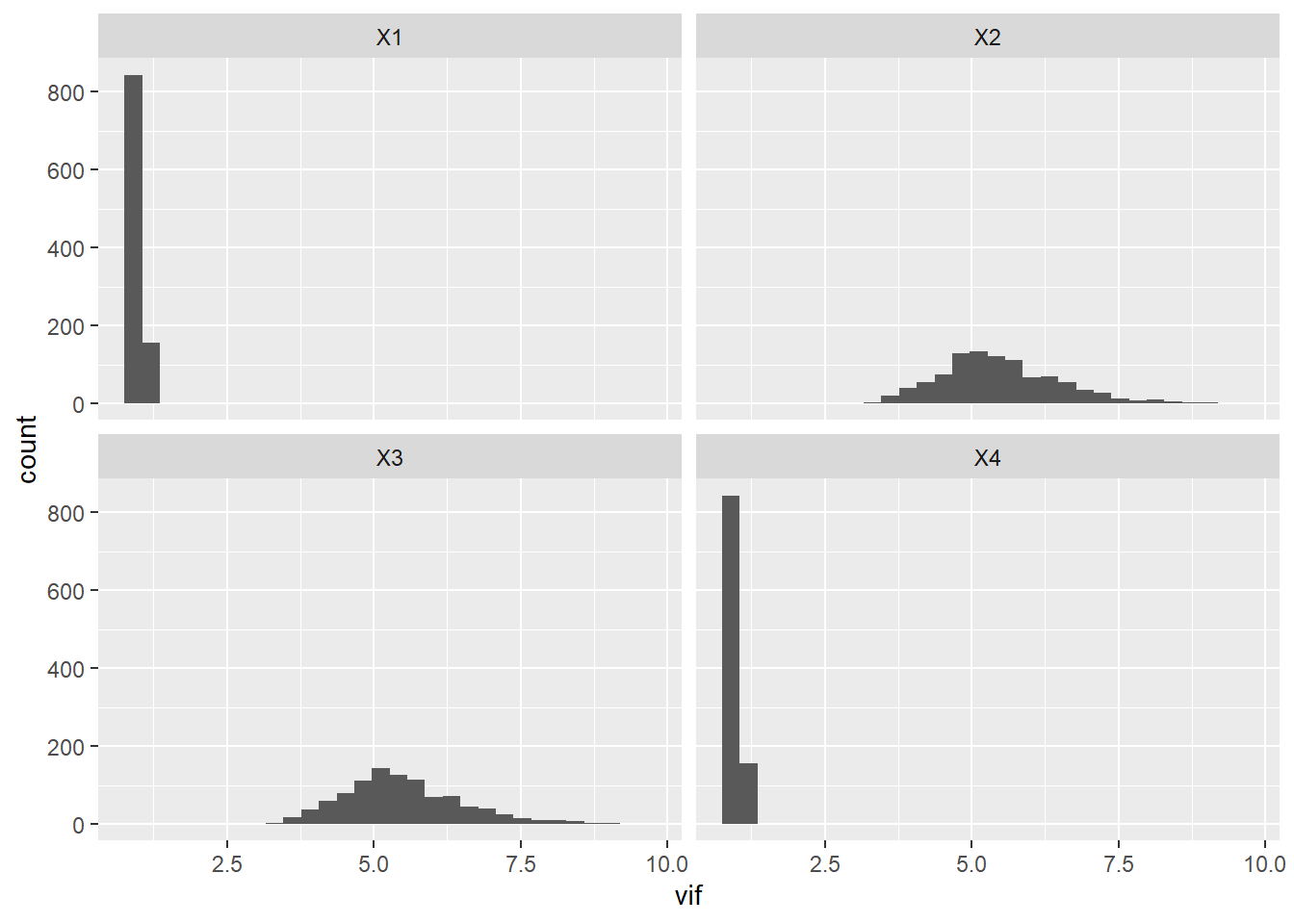# How big is too big: variance inflation factors

In a comment Jacob E. asked “Also, you mention using VIF for testing multi-collinearity. Is there a cutoff score you recommend for AIC?” I gave a snarky answer because I didn’t have a good answer. What would a good answer look like?

One way to proceed is to simulate data from the situation where the assumption is not violated, and look at the distribution of VIF scores.

library("tidyverse")
## -- Attaching packages --------------------------------------- tidyverse 1.3.1 --
## v ggplot2 3.3.3     v purrr   0.3.4
## v tibble  3.1.2     v dplyr   1.0.6
## v tidyr   1.1.3     v stringr 1.4.0
## v readr   1.4.0     v forcats 0.5.1
## -- Conflicts ------------------------------------------ tidyverse_conflicts() --
## x dplyr::lag()    masks stats::lag()
n <- 100
N <- 1000
make_data <- function(beta = c(0, 0.1, 1, 10), sigma = 1, residual_error = 1, n = 1){
if (is.null(dim(sigma))){
tmp <- sigma
sigma <- matrix(0, nrow = length(beta), ncol = length(beta))
diag(sigma) <- tmp
}
X <- MASS:::mvrnorm(n, mu = rep(0, length(beta)), Sigma = sigma)
Y <- X %*% beta + rnorm(n, mean = 0, sd = residual_error)
return(data.frame(Y, X))
}
#Test it out
test_df <- make_data(n = n)
ggplot(data = test_df,
mapping = aes(x = X4, y = Y, color = X3)) +
geom_point()OK, now generate a bunch of data sets, fit linear models to them, and get the VIF scores.

vif_null <- map(1:N, ~make_data(n = n)) %>%
map(~lm(Y~X1+X2+X3+X4, data = .)) %>%
map_dfr(car::vif) %>%
mutate(rep = 1:N) %>%
pivot_longer(cols = -rep, names_to = "variable",values_to = "vif")
ggplot(data = vif_null,
mapping = aes(x = vif)) +
geom_histogram() +
facet_wrap(~variable)
## stat_bin() using bins = 30. Pick better value with binwidth.OK, wow! Didn’t expect that – if there is NO correlation between the predictor variables then VIF rarely exceeds 1.2. Lets do an example where a couple of the variables are highly correlated.

sigma <- matrix(c(1, 0, 0, 0,
0, 1, -0.9, 0,
0, -0.9, 1, 0,
0, 0, 0, 1), nrow = 4, ncol = 4)
vif_bad <- map(1:N, ~make_data(n = n, sigma = sigma)) %>%
map(~lm(Y~X1+X2+X3+X4, data = .)) %>%
map_dfr(car::vif) %>%
mutate(rep = 1:N) %>%
pivot_longer(cols = -rep, names_to = "variable",values_to = "vif")
facet_wrap(~variable)
## stat_bin() using bins = 30. Pick better value with binwidth.